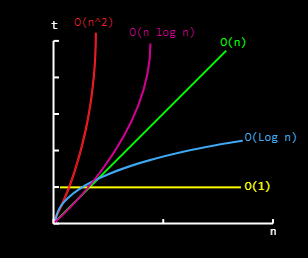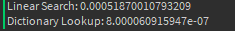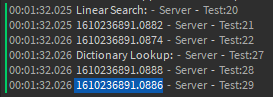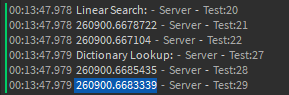# table.find(…) vs Key Indexing

This is an interesting problem I have been noticing throughout the community. I have been seeing a lot of people use `table.find` even when indexing a key in a dictionary was possible for them. In this article, I will be going over why that isn’t the most efficient solution and also a brief introduction to algorithms and time complexity.

# Ⅰ. Algorithms

What is an algorithm? Think of an algorithm as a set of instructions to achieve your result. A basic example would be baking a cake. Let’s look at some general steps on how to bake a cake.

1. Get ingredients
2. Mix
3. Pour batter into pan
4. Put in oven

That is a set of instructions to bake a cake. Though these steps could be more specific, we are only going to focus on the broader part. It seems that computers can also follow instructions we give to achieve our result the same way.

Now, let’s move away from the cake part and focus on giving a machine instructions. Let’s say we have an randomly-arranged array of numbers. How do we tell the computer to find the given number? Most of you reading this would probably say iterate over every number and check if it is the number we want. Ok, well let’s try to define that in pseudocode.

``````array= {1,4,2,0,9,10,3}
numberToFind = 2
foundNumber = false
for each number in array:
if number == numberToFind:
foundNumber = true
break

print(foundNumber)
``````

Now, as you can see from the pseudocode. We create an unsorted array that contains different numbers. We loop through each number in the array. If the number in the array is the number we want, then we change `foundNumber` to true and break the loop. Now let’s do the same thing but in Lua.

``````local numbers = {1,4,2,0,9,10,3}
local numberToFind = 2
local foundNumber = false
for _,number in ipairs(numbers) do
if number == numberToFind then
foundNumber = true
break
end
end
print(foundNumber)
``````

Congrats! We just created an algorithm to find a number. Now this leads us into a few questions? How efficient is our algorithm? Was there any other possible solution? How do we measure the efficiency of an algorithm?

# ⅠⅠ. Algorithm Efficiency

We are going to dive a little deeper into learning about time complexity(I don’t want this article to focus too much on the math part, so I won’t be explaining how to measure the time complexity). Time Complexity describes how many operations an algorithm has to do based on the input given.

It all starts with Big O Notation. If you have seen people say things like “Hey, that algorithm is O(n)” or “The time complexity of the algorithm is O(n^2)”, you have probably wondered what they mean. If you haven’t, don’t fret.

In Computer Science, we use Big O Notation to describe the performance of an algorithm(time complexity). Since machines are different and efficiency varies, we use Big O. O is basically a function and `n` is our input. If you go back to the algorithm we made in section one, `n` would the number of elements in the array.

Big O Notation tells us how many steps an algorithm has to take to achieve the result. It’s usually used to describe it in the worst-case scenario. What would be the worst case for the algorithm we made in section one? Well, let’s think about it. What if the number we wanted to find was at the very end of the array. How many steps or iterations will it take to find that number? It would take us `n` iterations or exactly the length of array.

Let’s say we added more numbers to the array. In the worse-case scenario, would it still take `n` number of steps? Yes, it would. Ok! Well, what about the best-case scenario? What if the number we were looking for was the first element of the array? How many iterations or steps would it take? It would take us `1` iteration.

Ok, we are starting to see the efficiency of this algorithm. So the algorithm we made in section one has a worst-case scenario of O(n) or a best-case scenario of Ω(1). We use the “Ω” or omega symbol to represent best-case scenario. It turns out we can actually graph this.I only want you to pay attention to the green and yellow line. `t` is how long the algorithm takes and `n` is how big our input is. As you can see, the green line is proportional or linear. For the green line, the time the algorithm takes is proportional to the input size. For the yellow line, no matter how large the input is, it will always take the same amount of time. We can call the green line a linear complexity and the yellow line a constant complexity.

Our algorithm we made is a linear complexity for the worst-case scenario. Now if we got lucky and the number were looking for was the first element, it would only take us 1 step. So our best-case scenario was a constant complexity.

# ⅠⅠⅠ. The Efficiency of table.find

If you take a look at the screenshot from Roblox API-Reference for table, you will see it says “A linear search algorithm is performed”. What is a linear search algorithm? Well, we implemented the same algorithm in section 1.

table.find works the same way. It has a worst-case scenario of O(n) and a best-case scenario of Ω(1). If you start thinking of large inputs to give table.find, you can see that the algorithm is not efficient. Again, we could get lucky and it could take us a few steps, but we could also be
extremely unlucky and the number was at the end of the array.

``````local array = {3, 2, 1, 5, 0, 4, 10, 32, 46, 21, 102, 59, 105}
table.find(array, 105) -- This takes us n iterations or for our case, exactly 13 iterations.
table.find(array, 3) -- This takes us 1 iteration.
``````

If you take a look at comments, you will see how many steps or iterations it would take to find the number.

# Ⅳ. table.find vs Key Indexing

Now in this section we are going to look at what’s more efficient. If your confused on what I mean by “key indexing”, it means to index into a dictionary using a key.

Now let’s think of a game scenario in where we would use table.find. How about a whitelist system? Only certain people can join your game(I know not the best scenario, but it works for now).

``````local whitelist = {  -- A table full of UserIds that are allowed to play your game.
12345678, 984210983, 741290,
8321038, 48103210, 48810321,
9321301, 83747310, 93931209,
8031203, 830821083, 1729321
}
if not table.find(whitelist, Player.UserId) then -- If it did not find the UserId in the table
Player:Kick("Not allowed to join the game.") -- Kick the player
end
end)
``````

Ok. We have a simple whitelist script. What if the player who joined had a UserId of `1729321`? It would take us `n` number of iterations to find it or the length of the table. As you can see, using table.find for this is not the most efficient way. What if we made the whitelist a dictionary using the UserId as the key?

``````local whitelist = {  -- A dictionary with UserIds as the key
["12345678"]=1, ["984210983"]=1, ["741290"]=1,
["8321038"]=1, ["48103210"]=1, ["48810321"]=1,
["9321301"]=1, ["83747310"]=1, ["93931209"]=1,
["8031203"]=1, ["830821083"]=1, ["1729321"]=1
}
if not whitelist[tostring(Player.UserId)] then -- If it did not find the UserId key
Player:Kick("Not allowed to join the game.") -- Kick the player
end
end)
``````

This is a lot more efficient than using table.find. We could have the value to be pretty much anything truthy. `1` is just short to type.

I hope this article helped you realize how there are better alternatives to table.find most of the time. For what table.find is meant to do, it is as efficient as it can be. If you notice any typos or mistakes, please let me know. As this is my first article on DevForum, it might not be the best. Thank you for reading.

44 Likes

You should also make a full post on why workspace is better than game.Workspace, it’s up to personal preference and just because you think it’s inefficient doesn’t mean it is.

4 Likes

Preoptimization is the root of all evil.

I see where your coming from, and the section on big O notation helped me immensely. But ultimately, the difference in efficiency probably wont make too much an impact in many cases.

Most applications are not dealing with massive datasets where a single item needs to be found. And in this case (with out knowing the specifics of the Key Value Pair implementation) I have to wonder if the proposed “optimized” solution you provided is ultimately the same linear search function under the hood.

I mean the key needs to be found somehow. Maybe an using the index would be faster (this is speculation and I’m not really sure).

Maybe you could offer some actual stats to back up your assertions? Use the roblox profiler to show us the difference in speed.

5 Likes

I did some benchmarking some time back and found what is implied by the implementations. `table.find` is just a linear search, and dictionary look-ups work with a hashmap.

That is to say, dictionary look-ups are orders of magnitude faster than are `table.find` calls, even when wrapped in a call of their own. The difference is negligible in smaller samples but I recall something of a ~x1000 speed up with dictionaries.

I would appreciate if the OP included their own benchmarks too, though.

10 Likes

Here are my benchmarks.

``````local dict = {}
local array = {}

for i = 1, 100000 do
table.insert(array, "n"..i)
dict["n"..i] = 1
end

local toFind = "n100000"

local t1 = os.clock()
if table.find(array, toFind) then
print("Linear Search: " .. os.clock()-t1)
end

local t2 = os.clock()
if dict[toFind] then
print("Dictionary Lookup: " .. os.clock()-t2)
end
``````Dictionary look-ups are much faster.

22 Likes

The difference is definitely easy to see however, these aren’t exactly humanly noticeable.

In an example of admin UserIds, unless you needed to store other data for that UserId (in the dictionary) then arrays & `table.find` suffice.

Programmers shouldn’t be steered away from methods due to micro-optimisations that can’t even be observed.

6 Likes

Yeah, it won’t make much difference with smaller inputs. I was just trying to get my point across to use dictionary lookups when you can and the basic idea of time complexity. This post can be a little confusing, but I do understand what you mean though.

1 Like

I mean dictionary indexing is easier to do and faster, why would someone want to do the harder and slower way?

1 Like

Keep in mind micro-optimization does not mean that ‘all optimization is bad’. Picking a method of access that is O(1) vs O(n) should not be overlooked, as it is a matter of writing overall performant code, not ‘micro-optimization’.

A good software developer will do this automatically, having developed a feel for where performance issues will cause problems. An inexperienced developer will not bother, misguidedly believing that a bit of fine tuning at a later stage will fix any problems.

11 Likes

Nice post. You’ll need to update the colors in part 2, though. Green is linear. Blue is logarithmic complexity.

Deciding not to write something more efficiently is a different issue than not knowing how or why one approach is better than another in a general sense. I think this is a helpful article for someone who may not know about the trade-offs they are dealing with concerning these two approaches and that they have a choice here that could make a substantial difference in the performance of their code in certain circumstances.

Using `table.find` is like having a row of mailboxes and looking through each one in turn to find your mail. With key indexing you know your mail is in box #5 (or whatever). If you only have a few boxes to look through, then it won’t make much difference which approach you choose. If you have thousands+ of boxes, looking through each one for your mail when you could just go directly to the box where it resides is silly, and slow.

These two approaches are meant for different problems. The keys are for rapid retrieval when you know the location (index) of the value you want. The table.find is for when you don’t know where a value is located (if it’s in the dict at all). For what it’s meant to do, table.find is efficient. There’s rarely ever a good reason to not use keys when you can organize data in a way that supports that approach.

6 Likes

There’s 0 point in an article like that.

The global variable `workspace` returns a direct reference to workspace, while `game.Workspace` will index “Workspace”. It’s better to just use the global variable, as you get a direct reference to Workspace.

3 Likes

Lookup tables(hashtables/dictionaries) are very cool and can replace table.find() easily, ofc u’d have to switch from arrays.

2 Likes

No. That is a bad justification.

2 Likes

Just a quick nitpick, hashtable look-up is not always O(1). In the case of chaining, which lua’s table support, sometimes the lookup has an average complexity of O(n) since it would need to search through a linked list. I’m not sure what hashing algorithm lua uses, but I doubt it’s anything special that prevents common collisions.

2 Likes

Your justification for the `workspace` global being bad practice is because it’s “inconsistent”.

But the thing is, the two are equivalent.

``````local WorkspaceService = game:GetService("Workspace")

print(WorkspaceService == workspace) --> true

local WorkspaceService = game:GetService("Workspace")

if WorkspaceService == workspace then
print("They are equivalent, no difference between them!") --> this is where it goes
else
print("They are not equivalent, there is a difference!")
end
``````

You can run this code, and it returns `true`, there is no difference, stop saying it’s bad practice to use the global.

2 Likes

This isn’t the place to discuss this. The thread I linked is. Please read my post thoroughly, as I provide a link with the Roblox style guidelines which directly contradict what you are saying. I’m not sure how much more clear it can get than Roblox’s own Lua guidelines.

4 Likes

Also by the way if this wasn’t mentioned Indexing can have hash collisions in, frequency of these collisions are determined by how good the hash function is.

So dictionary indexing be really bad if the hash function is bad. However most of the time dictionary indexing is 0(1) but at worst case dictionary indexing can be 0(something) since the hash function has to retry until the collision is resolved.

Dictiionaries use arrays undeaneath, and map a string to an array index.

This is why array indexing is also o(1).

2 Likes

The hash collisions in Lua are handled by making the buckets a linked list iirc. If a key gets mapped to the same bucket as another key, it gets added to the linked list. This does slow down search if the hash function fails to distribute keys evenly. Worse case scenario, it takes O(n) if all keys are mapped to the same bucket. But hashtables will also grow in size when the hash function seems to be inefficient.

2 Likes

I try your test by my way and i get this.

I don’t trust much in subtraction when it comes to functions like os.clock () or tick () so I will directly draw the differences

The difference:Linear search: 0.00080013275
Dictionary look-Ups: 0.00020003318
difference between both:0.00060009957

Conclusion: Dictionary is faster than Table.Find

Edit: I used tick()
By clock.os isLinear Seach: 0.00076819999
Dictionary lookup: 0.00020960002
difference between both = 0.00055859997

2 Likes

Your point is valid, although there’s SPACE complexity to consider as well. That isn’t strictly relevant here, but I mention it anyway.

For another concrete example to go with your whitelist, here’s my solution to both parts of this particular advent of code puzzle:

``````local input = "362981754"
local cups = {}

for c in input:gmatch(".") do
cups[#cups + 1] = tonumber(c)
end

local function doJob(iterations, length)
local lookup = {}
local cup = {n = cups}
lookup[cups] = cup
local temp = cup
for i = 2, length do
local n = {
n = cups[i] or i;
}
temp.next = n
lookup[n.n] = n
temp = n
end
temp.next = cup

for i = 1, iterations do
local frag = cup.next
cup.next = cup.next.next.next.next

local destn = (cup.n - 2)%length + 1
while destn == frag.n or destn == frag.next.n or destn == frag.next.next.n do
destn = (destn - 2)%length + 1
end

local dest = lookup[destn]
frag.next.next.next = dest.next
dest.next = frag

cup = cup.next
end

return lookup
end

io.write("Part 1:\t")
local pr = doJob(100, 9).next
for i = 1, 8 do
io.write(pr.n)
pr = pr.next
end
print()

local pr = doJob(10000000, 1000000).next
print("Part 2:", pr.n*pr.next.n)
``````

While you could get away with searching through the circular linked list I created, worst case scenario you search through 9,999,996 values for the second part each iteration.

By using additional memory to create that `lookup` Table (which isn’t a problem at all on my machine, since it’s only a few MB of pointers) I extremely significantly improve my algorithm’s performance.

For an contribution to the argument, though: there’s really no point either way if the Table you’re searching through is small enough and you aren’t searching more than a handful of times each frame.

Roblox games aren’t working on embedded systems with underpowered processors and RAM measured in KB; unless you’re running into serious performance issues, these types of optimizations are rarely worth considering.

3 Likes